# 题目描述（困难难度）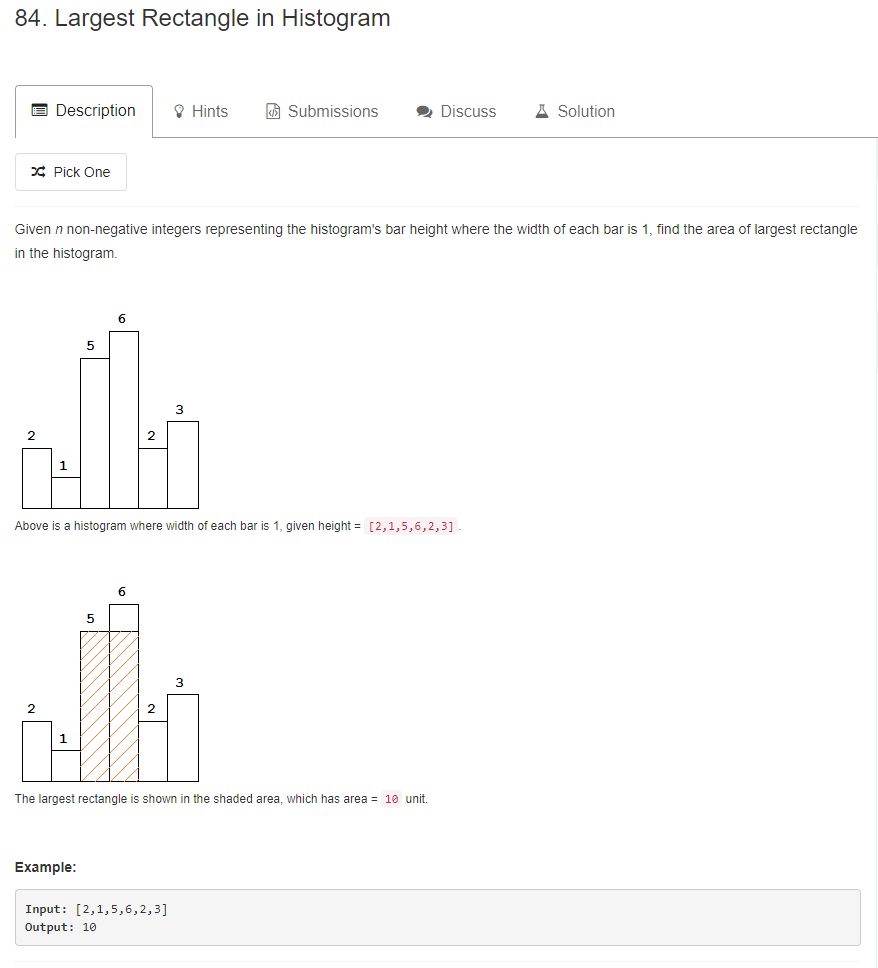# 解法一 暴力破解

public int largestRectangleArea(int[] heights) {
HashSet<Integer> heightsSet = new HashSet<Integer>();
//得到所有的高度，也就是去重。
for (int i = 0; i < heights.length; i++) {
}
int maxArea = 0;
//遍历每一个高度
for (int h : heightsSet) {
int width = 0;
int maxWidth = 1;
//找出连续的大于等于当前高度的柱形个数的最大值
for (int i = 0; i < heights.length; i++) {
if (heights[i] >= h) {
width++;
//出现小于当前高度的就归零，并且更新最大宽度
} else {
maxWidth = Math.max(width, maxWidth);
width = 0;
}
}
maxWidth = Math.max(width, maxWidth);
//更新最大区域的面积
maxArea = Math.max(maxArea, h * maxWidth);
}
return maxArea;
}


# 解法二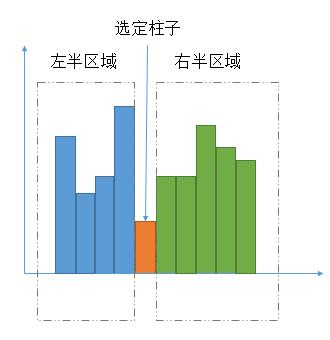1. 最大矩形区域在不包含选定柱子的左半区域当中。
2. 最大矩形区域在不包含选定柱子的右半区域当中。
3. 最大矩形区域包含选定柱子的区域。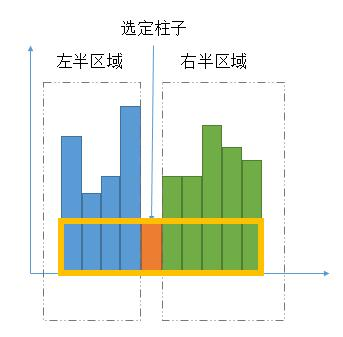T（n） = 2 * T（n / 2 ) + n。

T（n） = T（n - 1 ) + n。

T（n） = T（n - 1 ) + log（n）。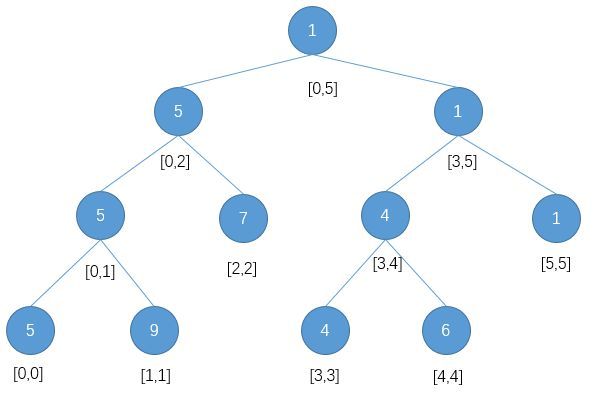class Node// 节点
{
int start;// 区间的左端点
int end;// 区间的右端点
int data;// 该区间的最小值
int index; // 该节点最小值对应数组的下标

public Node(int start, int end)// 构造方法中传入左端点和右端点
{
this.start = start;
this.end = end;
}

}

class SegmentTree {
private int[] base;;// 给定数组
Node[] nodes;// 存储线段树的数组

public SegmentTree(int[] nums) {
base = new int[nums.length];
for (int i = 0; i < nums.length; i++) {
base[i] = nums[i];
}
//需要 4n 的空间，上边链接给出了原因
nodes = new Node[base.length * 4];
}

public void build(int index)// 构造一颗线段树，传入下标
{
Node node = nodes[index];// 取出该下标下的节点
if (node == null) {// 根节点需要手动创建
nodes[index] = new Node(0, this.base.length - 1);
node = nodes[index];
}
if (node.start == node.end) {// 如果这个线段的左端点等于右端点则这个点是叶子节点
node.data = base[node.start];
node.index = node.start;
} else { // 否则递归构造左右子树
int mid = (node.start + node.end) >> 1;// 现在这个线段的中点
nodes[(index << 1) + 1] = new Node(node.start, mid);// 左孩子线段
nodes[(index << 1) + 2] = new Node(mid + 1, node.end);// 右孩子线段
build((index << 1) + 1);// 构造左孩子
build((index << 1) + 2);// 构造右孩子
if (nodes[(index << 1) + 1].data <= nodes[(index << 1) + 2].data) {
node.data = nodes[(index << 1) + 1].data;
node.index = nodes[(index << 1) + 1].index;
} else {
node.data = nodes[(index << 1) + 2].data;
node.index = nodes[(index << 1) + 2].index;
}
}
}

public Node query(int index, int start, int end) {
Node node = nodes[index];
if (node.start > end || node.end < start)
return null;// 当前区间和待查询区间没有交集，那么返回一个极大值
if (node.start >= start && node.end <= end)
return node;// 如果当前的区间被包含在待查询的区间内则返回当前区间的最小值
Node left = query((index << 1) + 1, start, end);
int dataLeft = left == null ? Integer.MAX_VALUE : left.data;
Node right = query((index << 1) + 2, start, end);
int dataRight = right == null ? Integer.MAX_VALUE : right.data;
return dataLeft <= dataRight ? left : right;

}
}
class Solution {
public int largestRectangleArea(int[] heights) {
if (heights.length == 0) {
return 0;
}
//构造线段树
SegmentTree tree = new SegmentTree(heights);
tree.build(0);
return getMaxArea(tree, 0, heights.length - 1, heights);
}
/**
*  查询某个区间的最小值
* @param tree 构造好的线段树
* @param start 待查询的区间的左端点
* @param end 待查询的区间的右端点
* @param heights 给定的数组
* @return 返回当前区间的矩形区域的最大值
*/
private int getMaxArea(SegmentTree tree, int start, int end, int[] heights) {
if (start == end) {
return heights[start];
}
//非法情况，返回一个最小值，防止影响正确的最大值
if (start > end) {
return Integer.MIN_VALUE;
}
//找出最小的柱子的下标
int min = tree.query(0, start, end).index;
//最大矩形区域包含选定柱子的区域。
int area1 = heights[min] * (end - start + 1);
//最大矩形区域在不包含选定柱子的左半区域。
int area2 = getMaxArea(tree, start, min - 1, heights);
//最大矩形区域在不包含选定柱子的右半区域。
int area3 = getMaxArea(tree, min + 1, end, heights);
//返回最大的情况
return Math.max(Math.max(area1, area2), area3);
}
}


# 解法三

T（n） = 2 * T（n / 2 ) + n。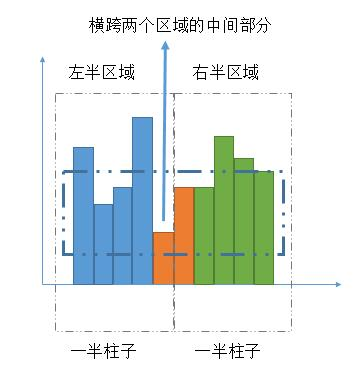1. 左半区域，含有一半柱子，当然如果总数是奇数个，这里会多一个。

2. 右半区域，含有一半柱子，当然如果总数是奇数个，这里会少一个。

3. 包含最中间柱子的部分，最大区域一定会包含橙色部分，这样才会横跨两个区域。

1. 初始的时候是两个柱子，记录此时的面积。

2. 然后加 1 个柱子，选取两边较高的柱子，然后计算此时的面积，更新最大区域面积。

3. 不停的重复过程 2 ，直到所有柱子遍历完
public int largestRectangleArea(int[] heights) {
if (heights.length == 0) {
return 0;
}
return getMaxArea(heights, 0, heights.length - 1);
}

private int getMaxArea(int[] heights, int left, int right) {
if (left == right) {
return heights[left];
}
int mid = left + (right - left) / 2;
//左半部分
int area1 = getMaxArea(heights, left, mid);
//右半部分
int area2 = getMaxArea(heights, mid + 1, right);
//中间区域
int area3 = getMidArea(heights, left, mid, right);
//选择最大的
return Math.max(Math.max(area1, area2), area3);
}

private int getMidArea(int[] heights, int left, int mid, int right) {
int i = mid;
int j = mid + 1;
int minH = Math.min(heights[i], heights[j]);
int area = minH * 2;
//向两端扩展
while (i >= left && j <= right) {
minH = Math.min(minH, Math.min(heights[i], heights[j]));
//更新最大面积
area = Math.max(area, minH * (j - i + 1));
if (i == left) {
j++;
} else if (j == right) {
i--;
//选择较高的柱子
} else if (heights[i - 1] >= heights[j + 1]) {
i--;
} else {
j++;

}
}
return area;
}


# 解法四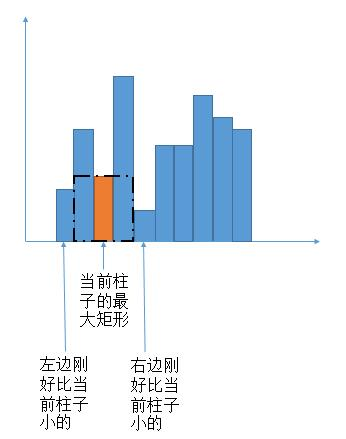leftLessMin = -1;//第一个柱子前边没有柱子，所以赋值为 -1，便于计算面积
for (int i = 1; i < heights.length; i++) {
int p = i - 1;
//p 一直减少，找到第一个比当前高度小的柱子就停止
while (p >= 0 && height[p] >= height[i]) {
p--;
}
leftLessMin[i] = p;
}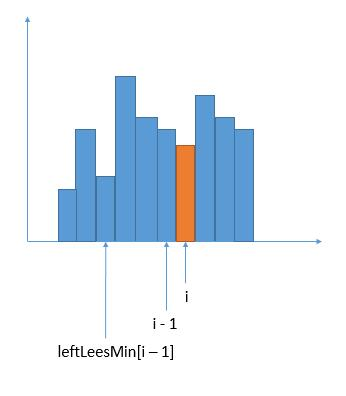int[] leftLessMin = new int[heights.length];
leftLessMin = -1;
for (int i = 1; i < heights.length; i++) {
int l = i - 1;
//当前柱子更小一些，进行左移
while (l >= 0 && heights[l] >= heights[i]) {
l = leftLessMin[l];
}
leftLessMin[i] = l;
}


public int largestRectangleArea(int[] heights) {
if (heights.length == 0) {
return 0;
}
//求每个柱子的左边第一个小的柱子的下标
int[] leftLessMin = new int[heights.length];
leftLessMin = -1;
for (int i = 1; i < heights.length; i++) {
int l = i - 1;
while (l >= 0 && heights[l] >= heights[i]) {
l = leftLessMin[l];
}
leftLessMin[i] = l;
}

//求每个柱子的右边第一个小的柱子的下标
int[] rightLessMin = new int[heights.length];
rightLessMin[heights.length - 1] = heights.length;
for (int i = heights.length - 2; i >= 0; i--) {
int r = i + 1;
while (r <= heights.length - 1 && heights[r] >= heights[i]) {
r = rightLessMin[r];
}
rightLessMin[i] = r;
}

//求包含每个柱子的矩形区域的最大面积，选出最大的
int maxArea = 0;
for (int i = 0; i < heights.length; i++) {
int area = (rightLessMin[i] - leftLessMin[i] - 1) * heights[i];
maxArea = Math.max(area, maxArea);
}
return maxArea;
}


# 解法五 栈

• 如果当前栈空，或者当前柱子大于栈顶柱子的高度，就将当前柱子的下标入栈
• 当前柱子的高度小于栈顶柱子的高度。那么就把栈顶柱子出栈，当做解法四中的当前要求面积的柱子。而右边第一个小于当前柱子的下标就是当前在遍历的柱子，左边第一个小于当前柱子的下标就是当前新的栈顶。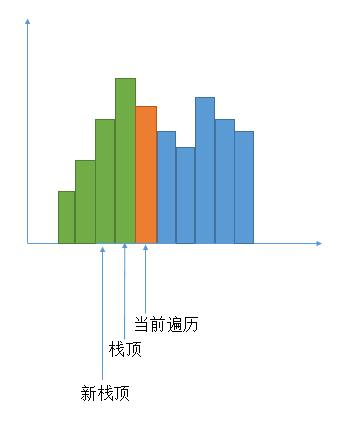public int largestRectangleArea(int[] heights) {
int maxArea = 0;
Stack<Integer> stack = new Stack<>();
int p = 0;
while (p < heights.length) {
//栈空入栈
if (stack.isEmpty()) {
stack.push(p);
p++;
} else {
int top = stack.peek();
//当前高度大于栈顶，入栈
if (heights[p] >= heights[top]) {
stack.push(p);
p++;
} else {
//保存栈顶高度
int height = heights[stack.pop()];
//左边第一个小于当前柱子的下标
int leftLessMin = stack.isEmpty() ? -1 : stack.peek();
//右边第一个小于当前柱子的下标
int RightLessMin = p;
//计算面积
int area = (RightLessMin - leftLessMin - 1) * height;
maxArea = Math.max(area, maxArea);
}
}
}
while (!stack.isEmpty()) {
//保存栈顶高度
int height = heights[stack.pop()];
//左边第一个小于当前柱子的下标
int leftLessMin = stack.isEmpty() ? -1 : stack.peek();
//右边没有小于当前高度的柱子，所以赋值为数组的长度便于计算
int RightLessMin = heights.length;
int area = (RightLessMin - leftLessMin - 1) * height;
maxArea = Math.max(area, maxArea);
}
return maxArea;
}